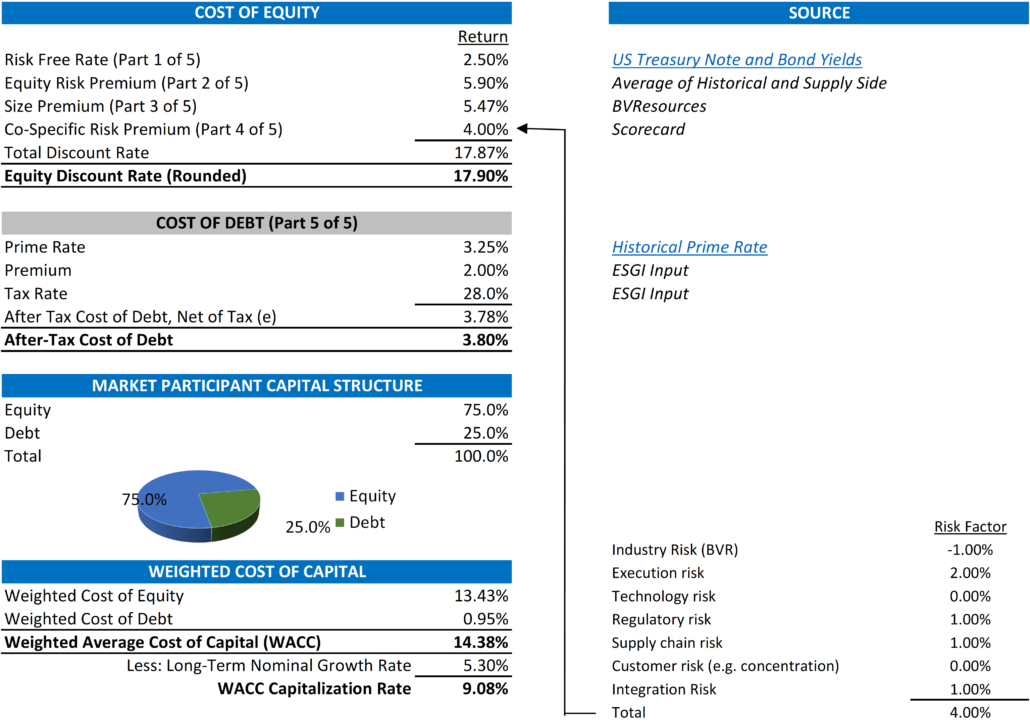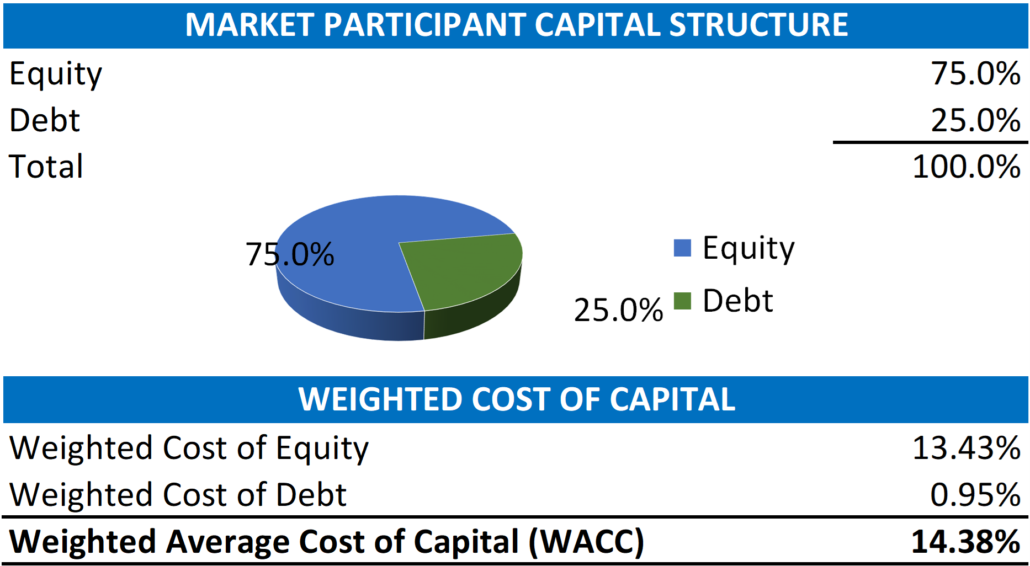# Understanding Discount Rates: The Cost of Debt and the Debt to Capital Ratio – Part 5 of 5

The first four parts of this five-part blog have dealt solely with the components of the buildup approach and the determination of a cost of equity. However, when determining a discount rate, it is important not to forget the ability to access debt. Each of the preceding parts of this discussion focuses on the cost of equity. This last part introduces the cost of debt and the debt to equity ratio in determining the weighted cost of capital (or “WACC”).

Build-Up Approach – Cost of Debt

In short, invested capital is equal to a Company’s capitalization or the total value of equity and debt. In our simple example above in determining the discount rate as the WACC, we are assuming debt is equal to bank debt only. While this assumption simplifies the determination of a discount rate, it also represents what would be needed in bank debt to settle the outstanding debt and obligations highlighted in the definition above.

The cost of debt is the interest rate (or the cost of borrowing debt) a Company pays on its debts. Similar to the build-up of the cost of equity, we start with a base rate of interest for a risk-free investment. While the cost of equity highlights this base rate as the cost of a US Treasury Note or Bond, the base cost of debt is identified by the prime lending rate (or “Prime Rate”). “The Prime Rate is the interest rate that commercial banks charge their most creditworthy corporate clients.”Similar to borrowing money to buy a house, the rate at which an individual or Company can borrow money depends on the collateral or the value of the asset used to secure a loan. Therefore, the rate at which a Company borrows money is based on the value of the assets that a bank can access if the Company defaults on the loan. How banks determine this collateral is a topic for another post. But banks are very similar to investors. The greater the risk, the greater return they require. As noted above, the “lever” to account for the risk is the premium a Company pays above the base Prime Rate. In our case, we have assumed a 2.0% (or 200 basis points where 100 basis points = 1%) as an example. Companies with more tangible assets should be able to get a lower rate. Companies with less tangible assets (think a start-up software company) will likely need to pay a greater premium. Of note, once this rate is determined, it is appropriate to use the after-tax cost of debt because a Company can utilize interest as an operating expense that lowers its tax liability.

Calculating WACC

So now that we have identified the approach to determining the cost of equity and the cost of debt and concluded that a discount rate is equal to the weighted average cost of capital or WACC, we come to the end of our discussion with one last important input to finally determine the WACC. This input is equal to the assumed amount of debt we apply to the capital structure (where the amount of equity is equal to 100% – the % amount of debt). Again, with a mortgage for a house, a lender usually requires at least 20% equity so the debt (or mortgage amount) is equal to 80%. For a business, these weightings have two key drivers, and the decision on which one to use is based on the scope of the valuation or analysis.

The two key drivers for a valuation are the % of the debt of the subject Company or the % of the debt that a market participant (or an investor in the industry) would likely have the ability to borrow. For the valuation of minority ownership (say a single share or 1.0% of a Company), the valuation is of a minority ownership interest. Because a minority owner has no control over how the Company spends (or borrows) money, we use the Company’s current % of debt to capital. For the valuation of a controlling ownership interest (greater than 50%), we assume that the potential buyer has the control to negotiate a deal with a bank based (like a mortgage) on how much equity is required to contribute to investment.

Once this % is determined, the calculation of the discount rate is a simple weighting exercise outlined below;What Does This All Mean?

The Cost of Debt and the amount of debt used in the calculation of WACC is both the last and for some companies the most important component of the discount rate. Debt helps mitigate overall risk by lowering the cost of an investment. It is a key component of corporate strategies that look to invest in projects with “cheap money” by leveraging their assets to grow their business. As such, it allows them to capture a more significant return on that investment.

We will present all five of these parts in their entirety so that you can understand every aspect of a discount rate.

Exit Strategies values control and minority ownership interests of private businesses for tax, financial reporting, strategic purposes. If you’d like help in this regard or have any related questions, you can reach  Joe Orlando, ASA at 503-925-5510 or jorlando@exitstrategiesgroup.com.